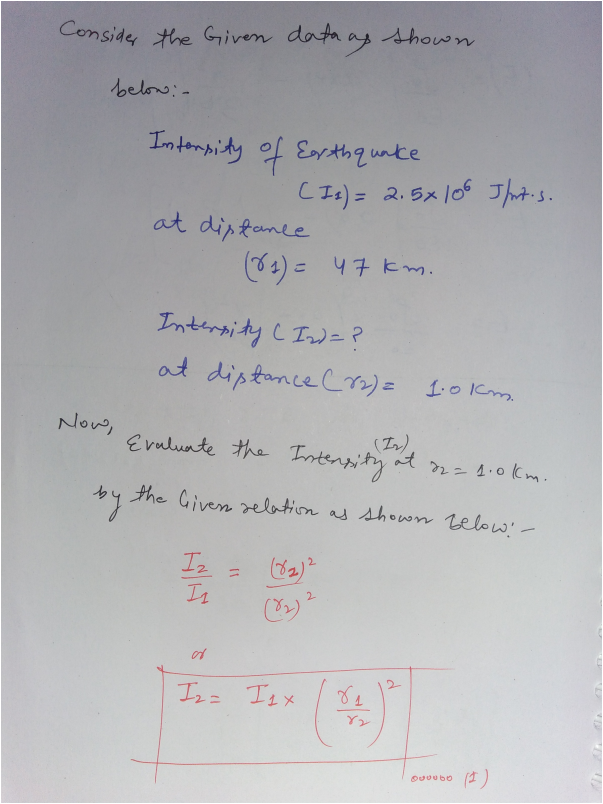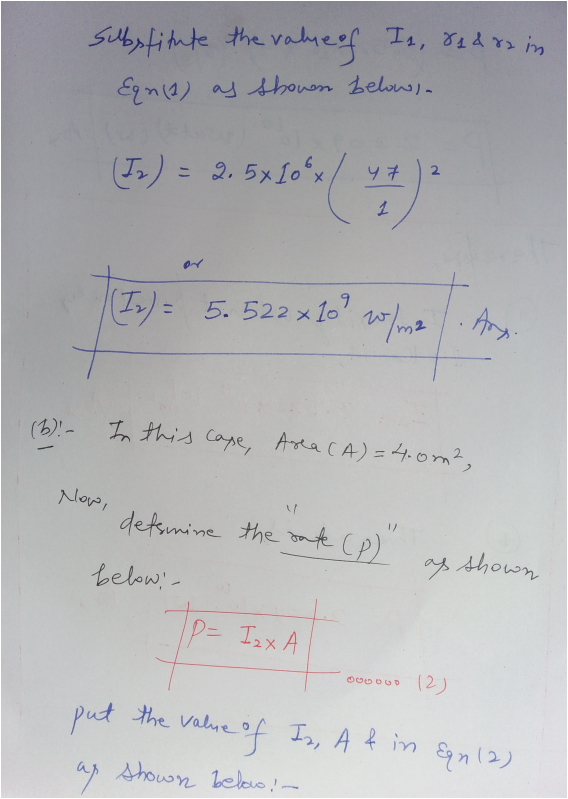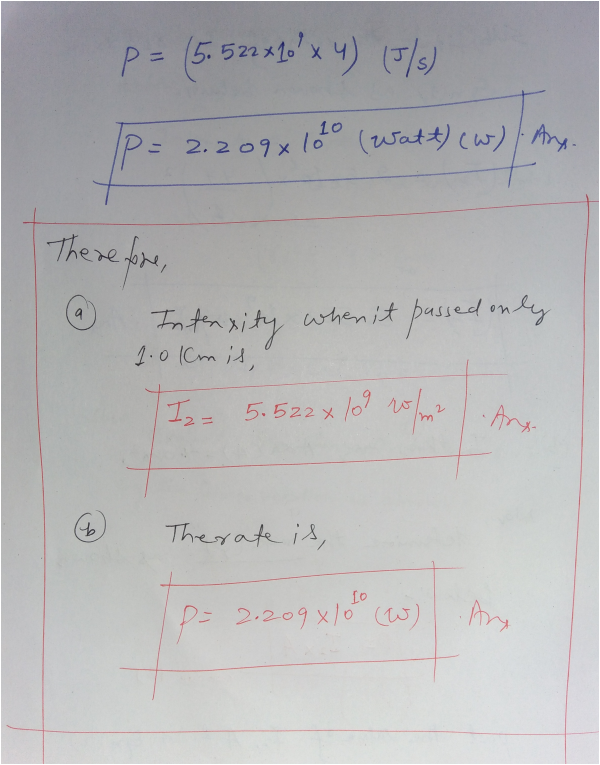In: Physics

# The intensity of an earthquake wave passing through the Earth is measured to be 2.5×106 J/(m2⋅s)...

The intensity of an earthquake wave passing through the Earth is measured to be 2.5×106 J/(m2⋅s) at a distance of 47 km from the source.

What was its intensity when it passed a point only 1.0 kmkm from the source?

Express your answer to two significant figures and include the appropriate units.

At what rate did energy pass through an area of 4.0 m2m2 at 1.0 kmkm ?

Express your answer to two significant figures and include the appropriate units.

## Solutions

##### Expert Solution## Related Solutions

##### The intensity of an earthquake wave passing through the Earth is measured to be 3.0 X...
The intensity of an earthquake wave passing through the Earth is measured to be 3.0 X 106 J/m2 s at a distance of 54 km from the source. (a) What was its intensity when it passed a point only 1.0 km from the source? (b) At what rate did energy pass through an area of 2.0m2 at 1.0 km?
##### The intensity of an earthquake wave passing through the Earth is measured to be 3.0 X...
The intensity of an earthquake wave passing through the Earth is measured to be 3.0 X 106 J/m2 s at a distance of 54 km from the source. (a) What was its intensity when it passed a point only 1.0 km from the source? (b) At what rate did energy pass through an area of 2.0m2 at 1.0 km?
##### An earthquake P wave traveling at 7.7km/s strikes a boundary within the Earth between two kinds...
An earthquake P wave traveling at 7.7km/s strikes a boundary within the Earth between two kinds of material. If it approaches the boundary at an incident angle of 44? and the angle of refraction is 33?, what is the speed in the second medium? Express your answer to two significant figures and include the appropriate units.
##### An earthquake emits both S-waves and P-waves which travel at different speeds through the Earth. A...
An earthquake emits both S-waves and P-waves which travel at different speeds through the Earth. A P=wave travels at 9000 m/s and an S-wave travels at 5000 m/s. If the P-waves are received at a seismic station 1 minute before an S-wave arrives, how far away is the earthquake center?
##### The P-wave from an earthquake arrives at a seismic station at 10:20 am and the S-wave...
The P-wave from an earthquake arrives at a seismic station at 10:20 am and the S-wave arrives at 10:25 am. Assuming the P-wave velocity is 5 km s−1, and that Poisson’s ratio is 0.25, compute the time at which the earthquake occurred and its epicentral distance (in degrees) from the seismic station.
##### The light at the Earth from a certain star has an intensity of about 71.5×10-8W/m2. If...
The light at the Earth from a certain star has an intensity of about 71.5×10-8W/m2. If the star emits radiation with the same power as our Sun, how far away is it from Earth? NOTE: Use 1000 W/m^2 as the intensity of the Sun at the Earth!
##### Radiation from the Sun reaching Earth (just outside the atmosphere) has an intensity of 1400 W/m2....
Radiation from the Sun reaching Earth (just outside the atmosphere) has an intensity of 1400 W/m2. What would the intensity be at Uranus? Assuming that Earth (and its atmosphere) behaves like a flat disk perpendicular to the Sun's rays and that all the incident energy is absorbed, calculate the force on Earth due to radiation pressure. What would it be for Uranus? What is the force on the Earth due to the sun's gravitational attraction? What is it for Uranus?
##### Radiation from the Sun reaching Earth (just outside the atmosphere) has an intensity of 1.35 kW/m2....
Radiation from the Sun reaching Earth (just outside the atmosphere) has an intensity of 1.35 kW/m2. (a) Assuming that Earth (and its atmosphere) behaves like a flat disk perpendicular to the Sun's rays and that all the incident energy is absorbed, calculate the force on Earth due to radiation pressure. (b) For comparison, calculate the force due to the Sun's gravitational attraction. Assume that the speed of light and Earth radius are 2.998 × 108 m/s and 6.37 thousand km...
##### Estimate the number of Solar neutrinos passing through a detector on the Earth in one second....
Estimate the number of Solar neutrinos passing through a detector on the Earth in one second. Assume that the detector has a surface area of 1.0 square meters and is aligned so that it faces directly towards the centre of the Sun. Assume that every neutrino travels without any change (i.e. none are absorbed or converted to a different particle). Also assume that the energy carried out of the Sun by the neutrinos is negligible
##### A polarized light wave passes through a polarizing filter which reduced the intensity of the light...
A polarized light wave passes through a polarizing filter which reduced the intensity of the light to 25% of the incident intensity. What would the intensity become if the incident light is unpolarized? PLEASE EXPLAIN :))